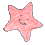### XOR swap algorithm

Did you know that you can swap the value of 2 variables without an auxiliary one? The most commonly used way is as follows:

```public static void main(String[] args) {
int x = 1;
int y = 2;
System.out.println("Before swap: X = " + x + ", Y = " + y);

int aux = x;
x = y;
y = aux;

System.out.println("After swap: X = " + x + ", Y = " + y);
}
```

But you actually don't need that aux variable:

```public static void main(String[] args) {
int x = 1;
int y = 2;
System.out.println("Before swap: X = " + x + ", Y = " + y);

x = x ^ y;
y = x ^ y;
x = x ^ y;
System.out.println("After swap: X = " + x + ", Y = " + y);
}
```

This method is known as the XOR swap algorithm.

1.Why not use the following notation? It's more concise.

x ^= y;
y ^= x;
x ^= y;

2.Yes, you're right, it's more concise but IMHO slightly less readable :)

3.Another method I found out, perhaps you could compare both approaches?

a += b;
b = a - b;
a += -b;

Also, the xor swap fails when x equals y, whilst this approach doesn't. That's one clear advantage.
However, I don't know how they compare in speed and on a low level; I guess it'd be an interesting extension to your article.

4.The XOR swap algorithm works fine when x = y, you might have tested wrong.

About your algorithm, yes, it works, but it is most likely to be slower than XOR algorithm. Logical operations are ALU's fastest, and XOR is one of them. Also in your algorithm you're doing more operations, even if you write them in one line: a += -b (here you got one unary operator then a binary one).

5.From wikipedia: "[...]if the addresses are equal, the algorithm will fold to a triple *x ^= *x resulting in zero."

Very interesting to know about ALU, though. I'm reading more on it right now.

6.You can try yourself and see that's it's working even if x = y, for example:

x = 1
y = 1

x = x ^ y (x = 0; y = 1)
y = x ^ y (x = 0; y = 1)
x = x ^ y (x = 1; y = 1)

7.You are actually correct, I should have tested it.

I believe you will find this interesting: https://rbt.asia/g/thread/32344720